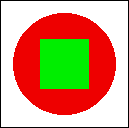## Quiz Number 5

This is the fifth quiz given in CPSC 124: Introductory Programming, Fall 1996. See the information page for that course for more information.

Question 1: One of the main classes in the AWT is the Component class. What is meant by a component? What are some examples?

Answer: A Component represents a visual component of the computer's graphical user interface. Components can be added to "containers," such as applets and frames. Examples of Components are Buttons, TextFields, and Canvases.

Question 2: An applet can define a method

public boolean mouseDown(Event evt, int x, int y)

What is the purpose of such a routine, and what is the meaning of the parameters x and y?

Answer: When the user clicks the mouse on the applet, the system will call the mouseDown() routine. The programmer can write such a routine to specify what should happen in response to the user's clicks. The parameters x and y are the horizontal and vertical coordinates of the point in the applet where the user clicked.

Question 3: Suppose you would like an applet that displays a green square inside a red circle, as illustrated. Write a paint() method that will draw the image.Answer: (The size of the square and circle are not specified in the problem, so any size would be acceptable, as long as the square is in the middle of the circle.)

```            public void paint(Graphics g) {
g.setColor(Color.red);
g.fillOval(10,10,80,80);
g.setColor(Color.green);
g.fillRect(30,30,30,30);
}
```

Question 4: In labs, you have been using the StatCalc class as an example. It includes the methods enter(double), getCount(), getMean(), and getSD(). Let's say I am curious to know the mean and the standard deviation of the 100 numbers 12, 22, 32,..., 1002. Write a main program that will use a StatCalc object to find out. Print out the mean and the standard deviation on the console.

Answer: (Recall that if stats is a StatCalc object, then the method stats.enter(num) method can be used to enter the number, num, into the objects data set. The method stats.getMean() returns the average of all the numbers that have been entered, and stats.getSD() returns the standard deviation of all the numbers.)

```               class QuizProgram {
public static void main(String[] args) {
Console console = new Console();
StatCalc stats = new StatCalc();
for (int i = 1; i <= 100; i++)
StatCalc.enter(i*i);
console.putln("The average is " + stats.getMean() );
console.putln("The standard deviation is " + stats.getSD() );
}
}
```

David Eck